Next: Solution: radial velocity , Up: 3.7 - Simple Potential Previous: Solution: Net outward flux

## 2D point vortex

Another particular solution: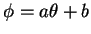(verify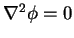except at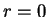)

Potential function for a point vortex of circulation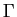at:

Stream function:

1.
Question: Evaluate the radial velocity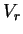, the tangential velocity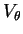and the vorticity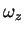.

(a)
Hint: velocity vector in polar coordinates (use the keyword utility: coordinate system - velocity vector).

(b)
Hint: write the vorticity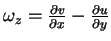in polar coordinates.

2.
Question: Evaluate the circulationalong an arbitrary closed contour containing the 2D vortex.

(a)
Hint: deform the closed contour to a circle containing the 2D vortex at the origin.
(b)
Hint: evaluate the velocity tangent to the circle.
(c)
Hint: integrate the tangent velocity along the circle.

Keyword SearchNext: Solution: radial velocity , Up: 3.7 - Simple Potential Previous: Solution: Net outward flux